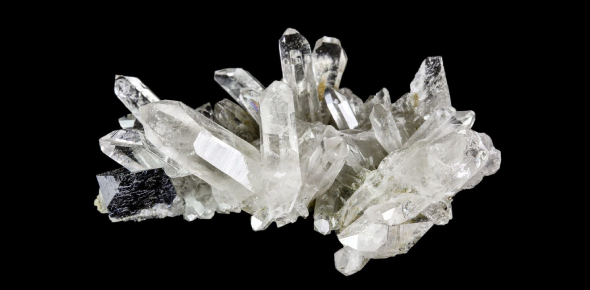# Ultimate Mineral Trivia Facts Quiz!

27 Questions | Total Attempts: 644Settings.

• 1.
The Most mined mineral in NC is:
• A.

Quartz

• B.

Feldspar

• C.

Gypsum

• D.

Silicon

• 2.
• A.

Living things

• B.

hydrogen oxides

• C.

Metallic ions

• D.

Non-living things

• 3.
Gold, Silver,  and Copper are examples of which mineral group?
• A.

Carbonates

• B.

Native elements

• C.

Metallic

• D.

Sulfides

• 4.
Check all that apply as classifications for a mineral.
• A.

Light in color

• B.

Inorganic solid

• C.

Crystal structure

• D.

Naturally occurring

• E.

Liquid at room temperature

• F.

Chemical composition

• 5.
If a mineral breaks along FLAT, SMOOTH surfaces it is exhibiting:
• A.

Fracture

• B.

Cleavage

• C.

Luster

• D.

Hardness

• 6.
Minerals that cool quickly will have _________ bonds and _______ sized crystal shapes.
• A.

Strong, large

• B.

Weak, small

• C.

Weak, large

• D.

Strong, small

• 7.
Quartz and Talc have the same density and streak.  What could you test to easily tell them apart?
• A.

Luster

• B.

Hardness

• C.

Color

• 8.
A large area that contains minerals that can be mined for profit.
• A.

Open pit

• B.

Over burden

• C.

Ore

• D.

Deposit

• 9.
Environmental results due to surface mining include the following:
• A.

Increase in erosion

• B.

Filter water ways

• C.

Ground and water contamination

• D.

Deforestzation

• 10.
The strongest type of mineral bonds are:
• A.

Van der walls

• B.

Ionic

• C.

Metallic

• D.

Covalent

• 11.
A mineral's density, chemical make up and crystal structure are dependent upon:
• A.

Arrangement of atoms

• B.

Rate of cooling

• C.

Hardness

• D.

Luster

• 12.
What are some special properties of quartz?
• A.

Small electrical currents

• B.

• C.

glows under ultraviolet light

• D.

Small vibrations

• E.

Magnetic

• 13.
An unknown mineral has a weight in air of 55g and a weight in water of 44g.  What is the specific gravity of this mineral?
• A.

11

• B.

5

• C.

97

• D.

3.5

• 14.
The powder of a mineral is called its:
• A.

Streak

• B.

Hardness

• C.

Luster

• D.

Cleavage

• 15.
Metalic bonds are:
• A.

Good electrical conductors

• B.

Can be pliable

• C.

Do not break easily

• D.

Are the weakest type of bond

• 16.
A minerals resistance to abrasion is known as:
• A.

Fracture

• B.

Cleavage

• C.

Hardness

• D.

Streak

• 17.
ON the Moh's Hardness scale, which is the softest and hardest mineral?
• A.

Talc, diamond

• B.

Mica, quartz

• C.

Talc, quartz

• D.

Mica, diamone

• 18.
The silicon-oxygen tetrahedron structure is made up of.....
• A.

1 oxygen and 4 silicon

• B.

4 oxygen and 4 silicon

• C.

4 of each oxygen and 1 hydrogen

• D.

1 silicon and 4 oxygen

• 19.
Which of the following is a non-silicate mineral?
• A.

Quartz

• B.

Diamond

• C.

Mica

• D.

Nickel

• 20.
What type of mining is a  shaft dug down to ore and then tunnels are dug either horizontal, vertical, or diagonal to access ore.
• A.

Strip

• B.

Shaft

• C.

Mountain top removal

• D.

Ope- pit

• 21.
Coal is a mineral.
• A.

True

• B.

False

• 22.
What is the NC gemstone?
• A.

Quartz

• B.

Emerald

• C.

Ruby

• D.

Azurite

• 23.
Within this mineral group, all minerals contain sulfides.
• A.

Carbonates

• B.

Sulfates

• C.

Oxides

• D.

Metalic

• 24.
This mineral is considered a PURE silicate mineral.
• A.

Calcite

• B.

Diamond

• C.

Quartz

• D.

Talc

• 25.
Quartz is used in which of the following items?
• A.

Watches

• B.

• C.

Telescopes

• D.

Microphones

• E.

Batteries

Related TopicsBack to top